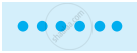Advertisement Remove all ads

# Introduction of Playing with Numbers

Advertisement Remove all ads

# Arranging the objects in rows and columns:

Ram has 6 marbles with him. He wants to arrange them in rows in such a way that each row has the same number of marbles. He arranges them in the following ways and matches the total number of marbles.

### Arrangement

1) 1 marble in each row
Number of rows = 6
Total number of marbles = 1 × 6 = 6.2) 3 marbles in each row
Number of rows = 2
Total number of marbles = 3 × 2 = 6.3) 6 marblesin a row.
Number of rows = 1
Total number of marbles = 6 × 1 = 6.4) 2 marbles in each row
Number of rows = 3
Total number of marbles = 2 × 3 = 6From these calculations Ramesh observes that 6 can be written as a productof two numbers in different ways as 6 = 1 × 6; 6 = 2 × 3; 6 = 3 × 2; 6 = 6 × 1

From 6 = 2 × 3 it can be said that 2 and 3 exactly divide 6. So, 2 and 3 are the exact divisors of 6. From the other product 6 = 1 × 6, the exact divisors of 6 are found to be 1 and 6. Thus, 1, 2, 3, and 6 are exact divisors of 6. They are called the factors of 6.

If you would like to contribute notes or other learning material, please submit them using the button below.
Advertisement Remove all ads
Share
Notifications

View all notifications

Forgot password?
Course